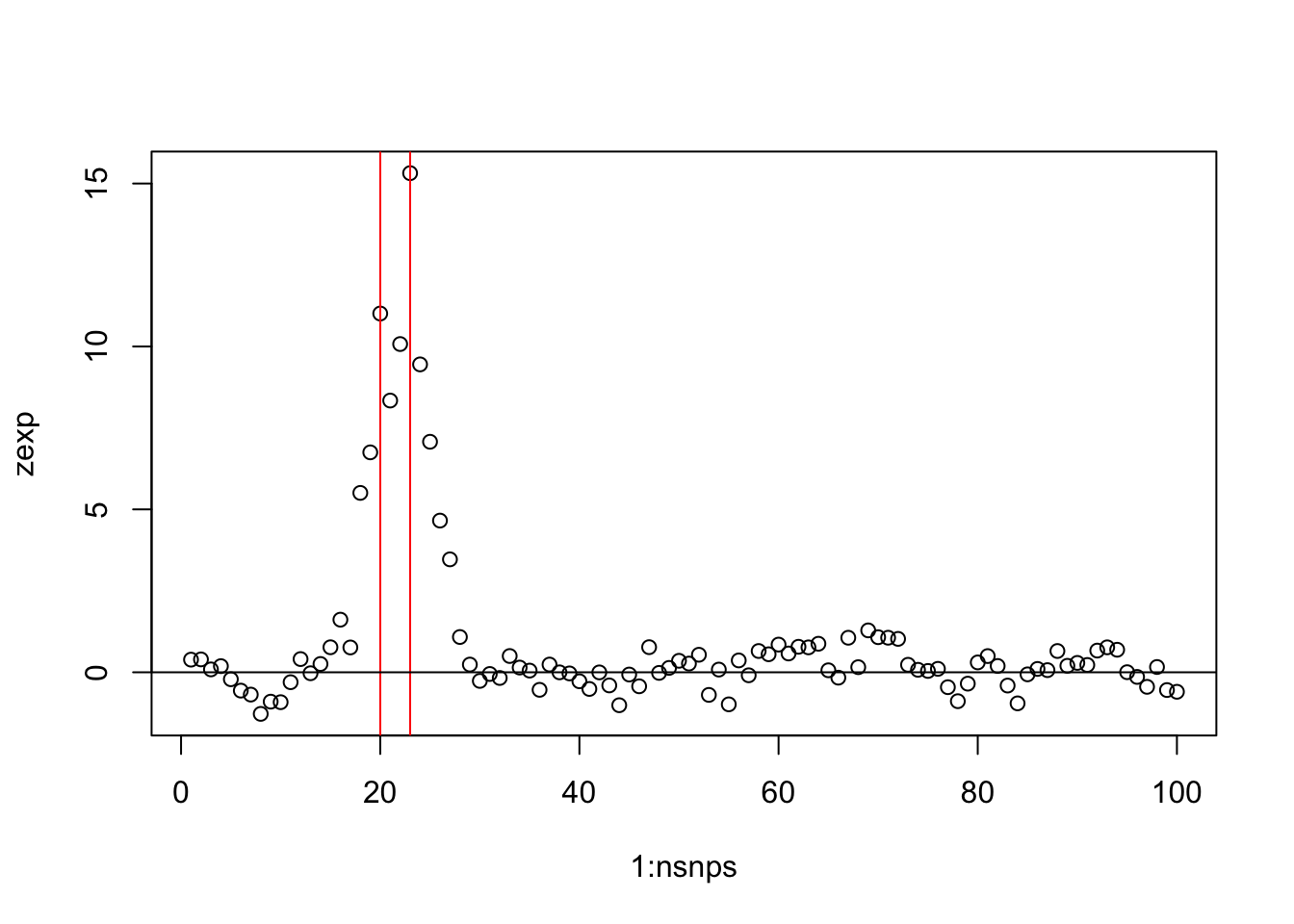``set.seed(20)``

## Functions

### 1. simref

If phased haplotypes are not avaliable, simref can be used to simulate some reference haplotypes. Lag is set to 5 due to the simple structure it provides for the variance/covariance matrix. This can be altered to allow for more or less linkage disequilibrium in simulations. The output is a \(nhaps*nsnps\) matrix of 0s and 1s with an extra columm, ‘Probability’, representing the probability of each haplotype.

``````# simref generates the reference haplotype
simref <- function(nsnps=100,nhaps=1000,lag=5) {
maf <- runif(nsnps+lag,0.05,0.5) # common SNPs
laghaps <- do.call("cbind", lapply(maf, function(f) rbinom(nhaps,1,f)))
haps <- laghaps[,1:nsnps]
for(j in 1:lag)
haps <- haps + laghaps[,(1:nsnps)+j]
haps <- round(haps/(lag+1))

snps <- colnames(haps) <- paste0("s",1:nsnps)
freq <- as.data.frame(haps+1)
freq\$Probability <- 1/nrow(freq)
freq
}``````

### 2. simdata

This function uses the output from simref and incorporates functions avaliable in the `simGWAS` and `coloc` packages to ultimately obtain the posterior probabilities of each SNP being the causal variant. The output is a list of nrep data frames. A flat prior of \(p1=1e-04\) is used.

``````# simdata generates the posterior probabilities for each SNP being causal
simdata <- function(freq,OR=1.5,nrep=100,N0=1000,N1=1000) {
snps <- colnames(freq)[-ncol(freq)] # snp names
CV=sample(snps,1) # choose a random CV

# calculate genotype probabilties at each SNP relative to the genotype at the CV
FP <- make_GenoProbList(snps=snps,W=CV,freq=freq)
zsim <- simulated_z_score(N0=N0, # number of controls
N1=N1, # number of cases
snps=snps,
W=CV, # causal variants, subset of snps
gamma.W=log(OR), # log odds ratios
freq=freq,# reference haplotypes
GenoProbList=FP,
nrep=nrep)
p <- 2*pnorm(-abs(zsim)) # generate p values from z values

# generate posterior probabilities using finemap.abf function
MAF <- colMeans(freq[,snps]-1) # minor allele frequencies
PP <- matrix(0,nrow(p),ncol(p)) # will hold the posterior probs
results <- lapply(1:nrep, function(i) {
tmp <- subset(finemap.abf(dataset=list(pvalues=p[i,], N=N0+N1, MAF=MAF,
s=N1/(N0+N1), type="cc"), p1=1e-04),snp!="null")
tmp\$SNP.PP <- tmp\$SNP.PP/sum(tmp\$SNP.PP) # replace post probs with the
# ratio of evidence for each variant being causal vrs all the others
colnames(tmp) <- sub("\\.\$","",colnames(tmp))
colnames(tmp) <- sub("SNP.PP","PP",colnames(tmp)) # clean up column names
tmp\$CV <- sub("SNP.","",tmp\$snp)==sub("s","",CV)
tmp[,c("snp","pvalues","MAF","PP","CV")] # include all the rows and the named columns
})
results
}``````

#### Example 1

This example illustrates the use of the `expected_z_score` and the `simulated_z_score` functions in the `simGWAS` package and is taken from the simGWAS vignette. Firstly, the expected z scores are calculated for each SNP, where \[z_E = E(Z).\]

``````nsnps <- 100
nhaps <- 1000
lag <- 5 # genotypes are correlated between neighbouring variants
maf <- runif(nsnps+lag,0.05,0.5) # common SNPs
laghaps <- do.call("cbind", lapply(maf, function(f) rbinom(nhaps,1,f)))
haps <- laghaps[,1:nsnps]
for(j in 1:lag)
haps <- haps + laghaps[,(1:nsnps)+j]
haps <- round(haps/matrix(apply(haps,2,max),nhaps,nsnps,byrow=TRUE))
snps <- colnames(haps) <- paste0("s",1:nsnps)
freq <- as.data.frame(haps+1)
freq\$Probability <- 1/nrow(freq)
sum(freq\$Probability)``````
``##  1``
``````CV=sample(snps[which(colMeans(haps)>0.1)],2) # choose two random SNPs as CVs
g1 <- c(1.4,1.2) # assign OR to these SNPs

FP <- make_GenoProbList(snps=snps,W=CV,freq=freq)
zexp <- expected_z_score(N0=10000, # number of controls
N1=10000, # number of cases
snps=snps, # column names in freq of SNPs for which Z scores should be generated
W=CV, # causal variants, subset of snps
gamma.W=log(g1), # odds ratios
freq=freq, # reference haplotypes
GenoProbList=FP) # FP above
plot(1:nsnps,zexp); abline(v=which(snps %in% CV),col="red"); abline(h=0)``````The simulated z scores are then calculated for each SNP where, \[z^* \sim N(z_E,\Sigma).\]

Note that the genotype probabilities do not need to be given as input to the function.

``````zsim <- simulated_z_score(N0=10000, # number of controls
N1=10000, # number of cases
snps=snps, # column names in freq of SNPs for which Z scores should be generated
W=CV, # causal variants, subset of snps
gamma.W=log(g1), # log odds ratios
freq=freq, # reference haplotypes
nrep=3)``````

The following plots show the simulated z scores plotted over the expected z scores.

``````par(mfcol=c(3,1))
for(i in 1:3) {
plot(1:nsnps,zexp,xlab="SNP",ylab="Z score"); abline(v=which(snps %in% CV),col="red"); abline(h=0)
title(main=paste("Replication",i))
points(1:nsnps,zsim[i,],col="blue",pch=2)
} ``````